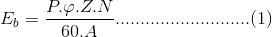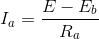# DC Motor or Direct Current Motor: What is it? (Diagram Included)

Contents

## What is a DC Motor?

An electric motor operated by DC (direct current) is known as a DC motor (unlike an induction motor that operates via an alternating current). A DC motor converts DC electrical energy into mechanical energy.

## Principle of DC Motor

When a current-carrying conductor is placed in a magnetic field, it experiences a torque and has a tendency to move.

In other words, when a magnetic field and an electric field interact, a mechanical force is produced. The DC motor or direct current motor works on that principle. This is known as motoring action.

The direction of rotation of this motor is given by Fleming’s left hand rule, which states that if the index finger, middle finger, and thumb of your left hand are extended mutually perpendicular to each other and if the index finger represents the direction of the magnetic field, middle finger indicates the direction of the current, then the thumb represents the direction in which force is experienced by the shaft of the DC motor.

Structurally and construction wise a direct current motor is exactly similar to a DC generator, but electrically it is just the opposite.

Here we unlike a generator we supply electrical energy to the input port and derive mechanical energy from the output port. We can represent it by the block diagram shown below.

In the DC motor above, the supply voltage E and current I is given to the electrical port or the input port and we derive the mechanical output i.e. torque T and speed ω from the mechanical port or output port.

Parameter K relates the input and output port variables of the direct current motor.So from the picture above, we can well understand that the motor is just the opposite phenomena of a DC generator, and we can derive both motoring and generating operation from the same machine by simply reversing the ports.

### Detailed Description of a DC Motor

To understand the DC motor in details lets consider the diagram below,

The circle in the center represents the direct current motor. On the circle, we draw the brushes. On the brushes, we connect the external terminals, through which we give the supply voltage.

On the mechanical terminal, we have a shaft coming out from the center of the armature, and the shaft couples to the mechanical load. On the supply terminals, we represent the armature resistance Ra in series.

Now, let the input voltage E, is applied across the brushes. Electric current which flows through the rotor armature via brushes, in presence of the magnetic field, produces a torque Tg. Due to this torque Tg the dc motor armature rotates.

As the armature conductors are carrying currents and the armature rotates inside the stator magnetic field, it also produces an emf Eb in a manner very similar to that of a generator.

The generated Emf Eb is directed opposite to the supplied voltage and is known as the back Emf, as it counters the forward voltage.
The back emf like in case of a generator is represented byWhere, P = no of poles
φ = flux per pole
Z= No. of conductors
A = No. of parallel paths
and N is the speed of the DC Motor.

So, from the above equation, we can see Eb is proportional to speed ‘N.’ That is whenever a direct current motor rotates; it results in the generation of back Emf. Now let’s represent the rotor speed by ω in rad/sec. So Eb is proportional to ω.

So, when the application of load reduces the speed of the motor, Eb decreases. Thus the voltage difference between the supply voltage and back emf increases that means E − Eb increases.

Due to this increased voltage difference, the armature current will increase and therefore torque, and hence speed increases. Thus a DC Motor is capable of maintaining the same speed under variable load.

Now armature current Ia is represented byNow at starting,speed ω = 0 so at starting Eb = 0.Now since the armature winding electrical resistance Ra is small, this motor has a very high starting current in the absence of back Emf. As a result, we need to use a starter for starting a DC Motor.
Now as the motor continues to rotate, the back emf starts being generated, and gradually the current decreases as the motor picks up speed.

### Types of DC Motors

Direct motors are classified according to the connection of the field winding to the armature.

There are 3 main types of DC Motors:

Want To Learn Faster? 🎓
Get electrical articles delivered to your inbox every week.
No credit card required—it’s 100% free.Electrical4U is dedicated to the teaching and sharing of all things related to electrical and electronics engineering.

### 2 thoughts on “DC Motor or Direct Current Motor: What is it? (Diagram Included)”

1.Thank you. Clear and informative.

•